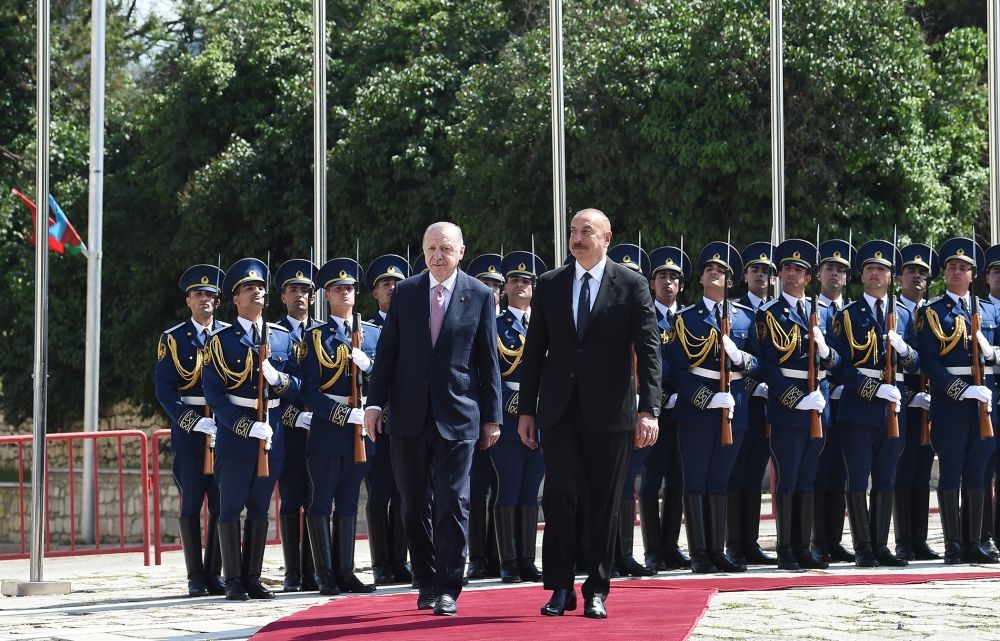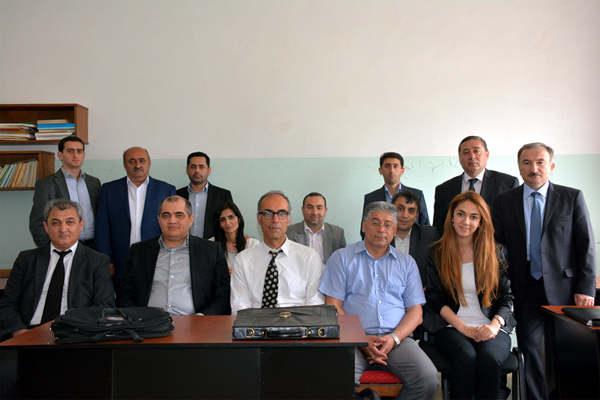Institute of Mathematics and Mechanics

## Karabakh news### “Musical heritage and Karabakh horses on Jidir Duzu plain” composition organized by Heydar Aliyev Foundation was presented in Shusha### Azerbaijan, Turkey signed Shusha Declaration on allied relations## Department of “Non-harmonic analysis”Head of the department: Prof. Bilal Bilalov Correspondent member of ANAS, Doctor of Sciences in Physics and Mathematics Tel: (+994 12)  5387250 E-mail: bilal.bilalov@imm.az , bilalov.bilal@gmail.com Number of employees: 24 Main Research Areas: Non-harmonic Fourier series; bases problems in linear topological spaces; basis properties of exponential systems in functional spaces; spectral properties of ordinary discrete  differential operators; frame theory-wavelet analysis and applications; financial (risk or actuary) mathematics, pattern recognition Main Scientific Results: Kostyuchenko problem from the spectral theory of differential operators is completely solved; classical Paley-Wiener and Bari theorems on bases are generalized with respect to the systems of elements  and the systems of subspaces; basicity criterion (Riesz basicity in the Hilbert case) for trigonometric systems in Lebesgue spaces with some asymptotics is found; the concept of  “b-basis” generated by some bilinear mapping which generalizes Schauder basis is introduced, classical facts about the theory of bases are extended to this case; major results on basicity for the systems with infinite defect in subspaces of Banach spaces are obtained; some Banach analogues of the Lax-Milgram theorem are proved; some complex analogues of well-known Stone-Weierstrass theorem  are proved and extended to the case of the space of piecewise continuous functions; basis properties of linear phase trigonometric systems are studied in generalized Lebesgue spaces; important results are obtained on basicity, equiconvergence, uniform and absolute convergence of series with respect to eigenfunctions and associated functions of differential operators; a classification of solutions of abstract differential equations with discontinuous coefficients in weighted Sobolev spaces is given, existence and uniqueness of solutions are proved; the concept of the regularity of the boundary conditions for discontinuous differential operators  is introduced  and the theorems  on a basicity  of the system of eigenfunctions and associated functions of a regular boundary value problems are proved; the results are obtained from the description of areas of fractional powers of discontinuous differential operators, for the study of basis properties of spectral problems containing the spectral parameter in the boundary conditions, in the spaces Lp + Cm and Lp abstract theorems are proved and  their application are given; a new method for obtaining  the bases in the direct sum of Banach spaces is introduced and their applications are given; basicity criterion in  for trigonometric systems with polynomial type phase is found. Basis properties of trigonometric type systems are studied in a Banach space of piecewise continuous functions; an abstract analogue of the Riemann problem is considered, Noetherness of this problem is studied and the obtained results are applied to basis-related problems.; the basicity of eigenfunctions of some discontinuous differential operator in Lebesgue spaces is studied; Some analogues of the well-known Kadets ¼-theorem with respect to the systems of exponent, cosines, sines  and some abstract analogues are obtained; abstract analogues of classical systems of power, sines and cosines are introduced,  a relationship between their basis  properties in Banach spaces is found; space of coefficients  generated by non-degenerate systems, transferred to different mathematical structures,  the generalizations of the theory of analytic functions  is given generated by  nilpotent and idempotent operators, the concept of corresponding bases is introduced; basis properties   of double systems consisting of generalized Faber polynomials with complex coefficients on Carleson curve is studied in Lebesgue spaces; Noetherness   perturbation of Hilbert and Banach frames is studied, the concept of t-frame generated by the Hilbert tensor product is introduced, and its   Noetherness   perturbation is studied; the basiscity  of the classical exponential systems in Morrey type spaces is proved; the basis properties of  perturbed trigonometric   systems in weighted generalized Lebesgue spaces are studied; the notion of statistical convergence  is transferred  to the   different mathematical structures,  the notion of μ-statistical convergence and μ-statistical  fundamentality  at the  point in a measurable space are introduced and their equivalence is proved; the notion of μ-statistical limits, μ-statistical completeness, μ-statistical fundamentality, μ-statistical equivalence of functions, μ-statistical continuity at infinity are introduced and some properties are studied; the basis  properties of trigonometric perturbed systems in  generalized and weighted generalized Lebesgue spaces are studied; signals of solar radiation (total, diffuse and reflected) are  processed by wavelet analysis.
Statistics on COVID-19 in Azerbaijan
• Infected with the virus

511974

• Healed

482578

• Newly infected

0

• Active patient

22549

• The state of death

6847

• Tested

5,081,920